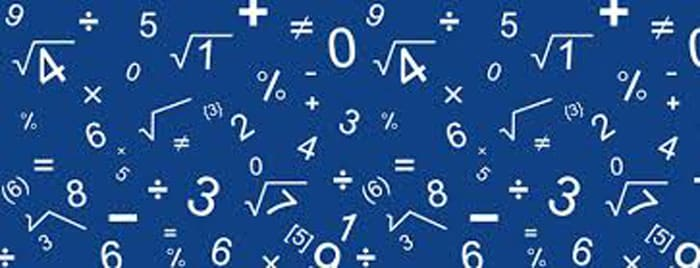# Quiz on Volume and Surface area## Surface Area & Volume Ratio multiple choice questions:

Question: The dimensions of a metallic cuboid are : 100 cm × 80 cm × 64 cm. It is melted and recast into a cube. Find the surface area of the cube
(a) 38400 cm2
(b) 12300 cm2
(c) 16400 cm2
(d) none of these

Ans. (a)

Question: The material of a cone is converted into the shape of a cylinder of equal radius. If the height of the cylinder is 5 cm, then the height of the cone is
(a) 10 cm
(b) 15 cm
(c) 18 cm
(d) 24 cm

Ans. (b)

Question: A solid sphere of radius 3 cm is melted and then cast into small spherical balls each of diameter 0.6 cm. Find the number of balls thus obtained
(a) 500
(b) 125
(c) 1000
(d) 375

Ans. (c)

Question: Three cubes each of side 5 cm, are joined end to end. Find the surface area of the resulting cuboid
(a) 350 cm2
(b) 175 cm2
(c) 125 cm2
(d) 50 cm2

Ans. (a)

Related: Lines and Angles MCQ

Question: A circus tent is cylindrical to a height of 4 m and conical above it. If its diameter is 105m and its slant height is 40 m, the total area of the canvas required in m2 is
(a) 1760
(b) 2640
(c) 3960
(d) 7920

Ans. (d)

Question: A conical vessel whose internal radius is 5 cm and height 24 cm is full of water. The water is emptied into a cylindrical vessel with internal radius 10 cms. Find the height to which the water rises.
(a)  4 cm
(b) 8 cm
(c) 16 cm
(d) 2 cm

Ans. (d)

Question: A solid is hemispherical at the bottom and conical above. If the surface area of the two parts is equal, then the ratio of its radius and the height of its conical part is
(a) 1 : 3
(b) 1 : √3
(c) 1 : 1
(d) √3 : 1

Ans. (b)

Question: The height of a cone is 30 cm. A small cone is cut off at the top by a plane parallel to the base. If its volume is 1/27 of the volume of the given cone, then the height above the base at which the section has been made is
(a) 10 cm
(b) 15 cm
(c) 20 cm
(d) 25 cm

Ans. (c)

Question: A metallic sphere of radius 10.5 cm is melted and then recast into small cones, each of radius 3.5 cm and height 3 cm. The number of such cones is
(a) 63
(b) 126
(c) 21
(d) 130

Ans. (b)

Question: A solid consists of a circular cylinder with an exact fitting right circular cone placed at the top. The height of the cone is h. If the total volume of the solid is 3 times the volume of the cone, then the height of the circular cylinder is
(a) 2h
(b) 2h/3
(c) 3h/2
(d) 4 h

Ans. (b)

Question: A right circular cone is of height 8.4 cm and the radius of its base is 2.1 cm. It is melted and recast into a sphere. Find the radius of the sphere
(a) 4.2 cm
(b) 2.1 cm
(c) 1.4 cm
(d) none of these

Ans. (b)

Question: A solid sphere of radius r is melted and cast into the shape of a solid cone of height r, the radius of the base of the cone is
(a) 2r
(b) 3r
(c) r
(d) 4r

Ans. (a)